Visitors Online: 77 | Wednesday 26th June 2019
 CBSE Guess > Papers > Question Papers > Class XII > 2005 > Maths > Outside Delhi Set-II MATHEMATICS 2005 (Set II—Outside Delhi)
Except for the following questions, all the remaining questions have been asked in Set I . SECTION - A  Q. 1. If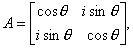then prove by Principle of mathematical Induction that, where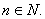Q. 2. Two balls are drawn at random with replacement from a box containing 10 black and 8 red. Find the probability that (i) both balls are red (ii) the first ball is black and the second is red (iii) one of them is black and the other red. Q. 10. Evaluate: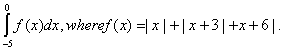Q. 14. Find the equation of the tangent to the curve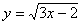which is parallel to the lineQ. 18. Using matrix method solve the following system of linear equations:SECTION - B Q. 19. In any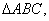prove that cos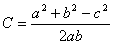with the help of vectors. Q. 22. A particle describes 650 m in 10 seconds and its velocity at the end of that is 80 m/sec. find its initial velocity and the acceleration. Q. 25. Find the equation of the plane passing through the pointsand perpendicular to the plane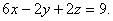SECTION - C  Q. 20. A bill for Rs. 1,50,000 was drawn on 14 th may,2003 for 5 months. It was discounted on 5 th August, 2003 at 7% per annum. Find the banker's discount- ted and the amount received by the holder of the bill. Q. 21. A big contains 1 white and 6 red balls. and a second bag contains 4 white and 3 red balls. One of the bags is picked up at random and a ball is randomly drawn from it, and is found to be white in colour. Find the probability that the drawn that the drawn ball was the first bag. Q. 25. A, B and C started a business by investing capital in the ratio of 3 : 4 : 5 : A and B respectively worked as Manager and Assistant Manager and were paid 15% and 10% respectively of the total profit for their services, the remaining profit was divided invested. If at the end of the year, A received Rs. 81,000, in all find the total profit of the businees and the share of B in all. Maths 2005 Question Papers Class XII Delhi Outside Delhi Compartment Delhi Compartment Outside Delhi Set I Set I Set I Set I Set II Set II Set II Set II Set III Set III CBSE 2005 Question Papers Class XII